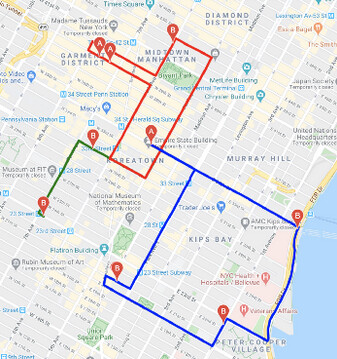# Plot multiple Routes [closed]

There is a nice example, how one can plot multiple Routes (e.g. A->B, C->D, …) using Google Maps API. The code is simple and straightforward (from here):

`for k in range(vehicle_count):`
`for i in range(customer_count):`
` for j in range(customer_count):`
` if i != j and pulp.value(x[i][j][k]) == 1:`
` layer.append(gmaps.directions.Directions(`
`(df.latitude[i],df.longitude[i]),`
` (df.latitude[j],df.longitude[j]),`
`mode='car',stroke_color=color_list[k],stroke_opacity=1.0, stroke_weight=5.0))`
`for i in range(len(layer)):`
`fig.add_layer(layer[i])`

The results are the plotted routes:Therefore, my question: Are there similar simple ways in Graphhopper for plotting multiple Trips (A->B, C->D, …)?

For JavaScript you can utilize our library.

See here for the demo: JavaScript examples for the GraphHopper Directions API

Thanks for the fast reply! (Should I close the topic?)

Sure, you can close the topic if this solved your issue